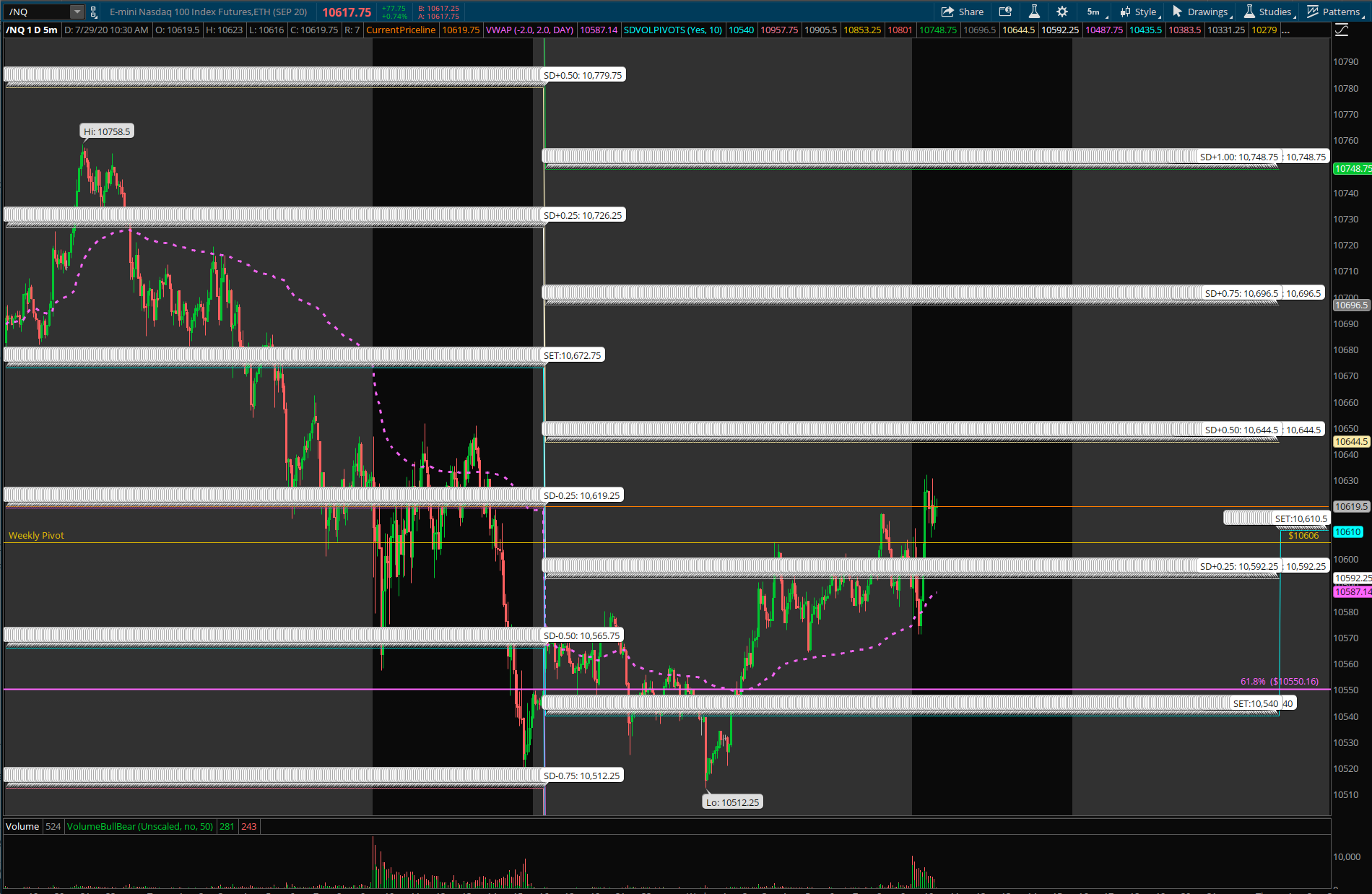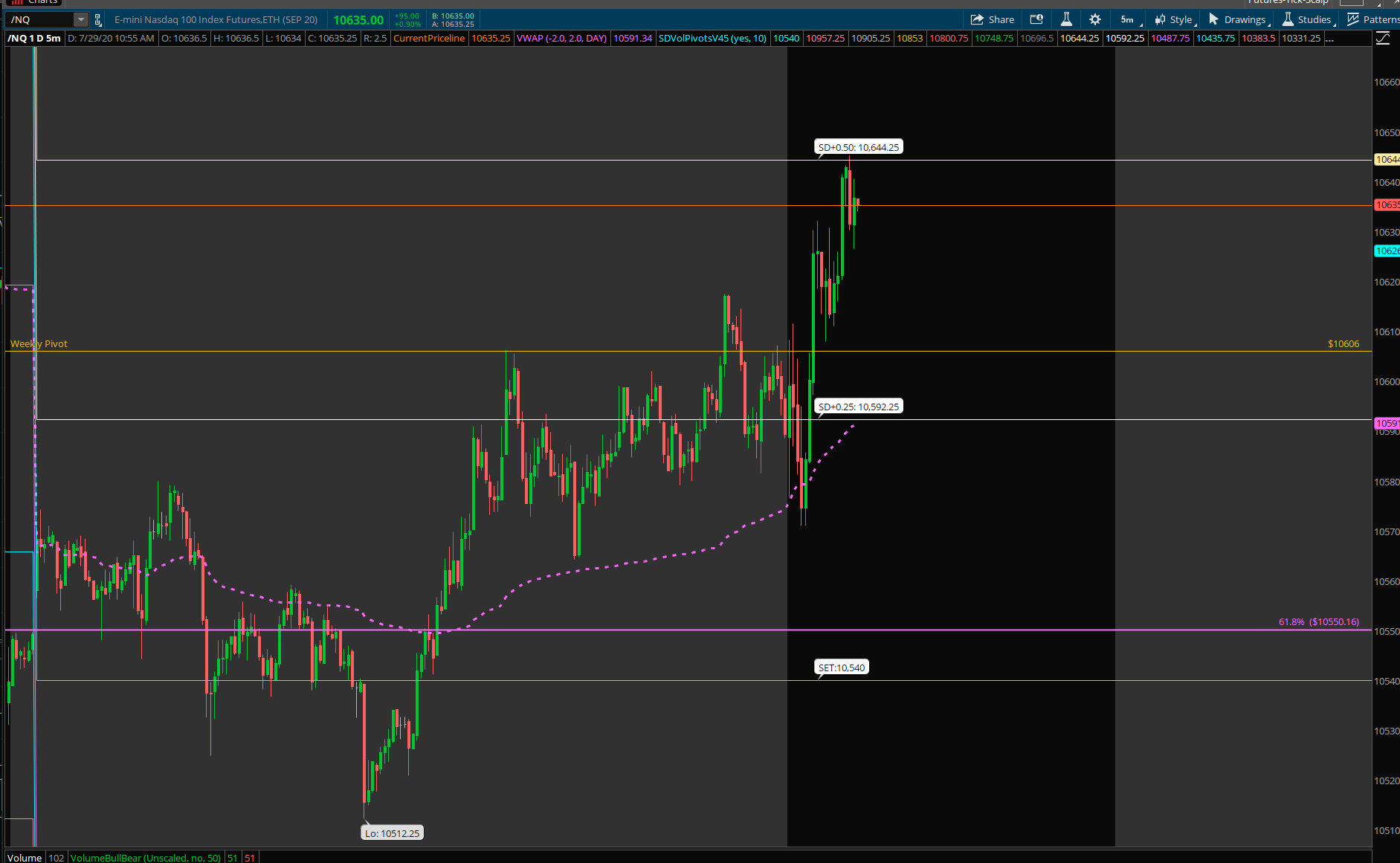# AddChartBubble() Not Working in ThinkorSwim

#### BillMurray

##### Member
Any help would be greatly appreciated figuring out why my chart bubbles won't display. Here's the thinkscript code I'm using.

Code:
``````### SD v4 (first versio in TOS)

# offset is the adjustment factor going from 'fair value' (??) to the /NQ contract.
# we will have to monitor this because it will probably change with DTE, interest rates, and any dividend that is payed out.
# as of 28 July 2020, offset of 9.25 seems to produce a result similar to v4 in pine.
def offset = 9.25;

# pulling in values for contract being traded and its volatility measure
def stl = close("/NQ", aggregationPeriod.DAY) - offset;
def iv = close("VXN", aggregationPeriod.DAY);

# variables for chart bubble management
input ShowBubbles = {default "Yes", "No"};#Hint ShowBubbles: Show/Hide chart bubbles
input ShiftBubble = 10;
def n1 = ShiftBubble+1;
def bar = BarNumber();

# vol math.
# logic works but can be re-written to appear more pseudocode-ish, for easier readability.
def b1 = iv / 100;
def b2 = b1 / sqrt(252);
def b3 = b2 * stl;

# the next two blocks can be rewritten as one (ie the math and rounding can be accomplished in a single 'plot' statement, but it was easier to write it this way for debugging while i was trying different inputs for 'stl', 'iv', and 'offset'.
plot std0 = stl;
def std1u = std0 + 2 * b3;
def std2u = std0 + 1.75 * b3;
def std3u = std0 + 1.5 * b3;
def std4u = std0 + 1.25 * b3;
def std5u = std0 + 1 * b3;
def std6u = std0 + 0.75 * b3;
def std7u = std0 + 0.5 * b3;
def std8u = std0 + 0.25 * b3;
def std9u = std0 + -0.25 * b3;
def std10u = std0 + -0.5 * b3;
def std11u = std0 + -0.75 * b3;
def std12u = std0 + -1 * b3;
def std13u = std0 + -1.25 * b3;
def std14u = std0 + -1.5 * b3;
def std15u = std0 + -1.75 * b3;
def std16u = std0 + -2 * b3;

plot std1 = Round(std1u/ TickSize(), 0) * TickSize();
plot std2 = Round(std2u/ TickSize(), 0) * TickSize();
plot std3 = Round(std3u/ TickSize(), 0) * TickSize();
plot std4 = Round(std4u/ TickSize(), 0) * TickSize();
plot std5 = Round(std5u/ TickSize(), 0) * TickSize();
plot std6 = Round(std6u/ TickSize(), 0) * TickSize();
plot std7 = Round(std7u/ TickSize(), 0) * TickSize();
plot std8 = Round(std8u/ TickSize(), 0) * TickSize();
plot std9 = Round(std9u/ TickSize(), 0) * TickSize();
plot std10 = Round(std10u/ TickSize(), 0) * TickSize();
plot std11 = Round(std11u/ TickSize(), 0) * TickSize();
plot std12 = Round(std12u/ TickSize(), 0) * TickSize();
plot std13 = Round(std13u/ TickSize(), 0) * TickSize();
plot std14 = Round(std14u/ TickSize(), 0) * TickSize();
plot std15 = Round(std15u/ TickSize(), 0) * TickSize();
plot std16 = Round(std16u/ TickSize(), 0) * TickSize();

# the condition ('cond') variables below were being used to debug the label statements.
# if cond is set to 'yes', then bubbles appear along the length of the chart.
# ideally, for each std0 increments, a single bubble would be positioned maybe 10 bars (?) after the current bar

def cond2 = ShowBubbles and isNaN(close[ShiftBubble]) and !isNaN(close[n1]) ;
def cond3 = barNumber() == close();
def cond = no;

# labels. stupid, stupid labels.

# more testing for bubbles. stupid, stupid bubbles. smdh.
AddChartBubble(bar == HighestAll(bar) and ShowBubbles, std0, concat("SET:", std0), Color.WHITE, yes);``````

Solution
Did it in a bit of a hurry, but something like this? Removed some code and changed a few things.

Code:
``````### SD v4 (first versio in TOS)

# offset is the adjustment factor going from 'fair value' (??) to the /NQ contract.
# we will have to monitor this because it will probably change with DTE, interest rates, and any dividend that is payed out.
# as of 28 July 2020, offset of 9.25 seems to produce a result similar to v4 in pine.
def offset = 9.25;

# pulling in values for contract being traded and its volatility measure
def stl = close("/NQ", aggregationPeriod.DAY) - offset;
def iv = close("VXN", aggregationPeriod.DAY);

# variables for chart bubble management
input ShowBubbles = yes; #Hint ShowBubbles: Show/Hide chart bubbles
input...``````
Code:
``````# the condition ('cond') variables below were being used to debug the label statements.
# if cond is set to 'yes', then bubbles appear along the length of the chart.
# ideally, for each std0 increments, a single bubble would be positioned maybe 10 bars (?) after the current bar

def cond2 = ShowBubbles and isNaN(close[ShiftBubble]) and !isNaN(close[n1]) ;
def cond3 = barNumber() == close();
def cond = no;``````

Perhaps try changing "def cond = no;" to "yes"?

Thanks Pensar! Now it looks like this. Chart bubbles showing, but that painting along the level? hmmmDid it in a bit of a hurry, but something like this? Removed some code and changed a few things.

Code:
``````### SD v4 (first versio in TOS)

# offset is the adjustment factor going from 'fair value' (??) to the /NQ contract.
# we will have to monitor this because it will probably change with DTE, interest rates, and any dividend that is payed out.
# as of 28 July 2020, offset of 9.25 seems to produce a result similar to v4 in pine.
def offset = 9.25;

# pulling in values for contract being traded and its volatility measure
def stl = close("/NQ", aggregationPeriod.DAY) - offset;
def iv = close("VXN", aggregationPeriod.DAY);

# variables for chart bubble management
input ShowBubbles = yes; #Hint ShowBubbles: Show/Hide chart bubbles
input ShiftBubble = 10;
def n1 = ShiftBubble+1;
def bar = BarNumber();

# vol math.
# logic works but can be re-written to appear more pseudocode-ish, for easier readability.
def b1 = iv / 100;
def b2 = b1 / sqrt(252);
def b3 = b2 * stl;

# the next two blocks can be rewritten as one (ie the math and rounding can be accomplished in a single 'plot' statement, but it was easier to write it this way for debugging while i was trying different inputs for 'stl', 'iv', and 'offset'.
plot std0 = stl;
def std1u = std0 + 2 * b3;
def std2u = std0 + 1.75 * b3;
def std3u = std0 + 1.5 * b3;
def std4u = std0 + 1.25 * b3;
def std5u = std0 + 1 * b3;
def std6u = std0 + 0.75 * b3;
def std7u = std0 + 0.5 * b3;
def std8u = std0 + 0.25 * b3;
def std9u = std0 + -0.25 * b3;
def std10u = std0 + -0.5 * b3;
def std11u = std0 + -0.75 * b3;
def std12u = std0 + -1 * b3;
def std13u = std0 + -1.25 * b3;
def std14u = std0 + -1.5 * b3;
def std15u = std0 + -1.75 * b3;
def std16u = std0 + -2 * b3;

plot std1 = Round(std1u/ TickSize(), 0) * TickSize();
plot std2 = Round(std2u/ TickSize(), 0) * TickSize();
plot std3 = Round(std3u/ TickSize(), 0) * TickSize();
plot std4 = Round(std4u/ TickSize(), 0) * TickSize();
plot std5 = Round(std5u/ TickSize(), 0) * TickSize();
plot std6 = Round(std6u/ TickSize(), 0) * TickSize();
plot std7 = Round(std7u/ TickSize(), 0) * TickSize();
plot std8 = Round(std8u/ TickSize(), 0) * TickSize();
plot std9 = Round(std9u/ TickSize(), 0) * TickSize();
plot std10 = Round(std10u/ TickSize(), 0) * TickSize();
plot std11 = Round(std11u/ TickSize(), 0) * TickSize();
plot std12 = Round(std12u/ TickSize(), 0) * TickSize();
plot std13 = Round(std13u/ TickSize(), 0) * TickSize();
plot std14 = Round(std14u/ TickSize(), 0) * TickSize();
plot std15 = Round(std15u/ TickSize(), 0) * TickSize();
plot std16 = Round(std16u/ TickSize(), 0) * TickSize();

# ideally, for each std0 increments, a single bubble would be positioned maybe 10 bars (?) after the current bar

def cond2 = ShowBubbles and !isNaN(close[-ShiftBubble]) and isNaN(close[-n1]);

# labels. stupid, stupid labels.

# more testing for bubbles. stupid, stupid bubbles. smdh.
AddChartBubble(bar == HighestAll(bar) and ShowBubbles, std0, concat("SET:", std0), Color.WHITE, yes);``````

•RickAns and BillMurray
Thanks!•Pensar

### Not the exact question you're looking for?

87k+ Posts
291 Online## The Market Trading Game Changer

Join 2,500+ subscribers inside the useThinkScript VIP Membership Club
• Exclusive indicators
• Proven strategies & setups
• Private Discord community
• Exclusive members-only content
• 1 full year of unlimited support

What is useThinkScript?

useThinkScript is the #1 community of stock market investors using indicators and other tools to power their trading strategies. Traders of all skill levels use our forums to learn about scripting and indicators, help each other, and discover new ways to gain an edge in the markets.

How do I get started?

We get it. Our forum can be intimidating, if not overwhelming. With thousands of topics, tens of thousands of posts, our community has created an incredibly deep knowledge base for stock traders. No one can ever exhaust every resource provided on our site.

If you are new, or just looking for guidance, here are some helpful links to get you started.

What are the benefits of VIP Membership?